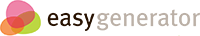# Solving Equations

## Key Vocabulary

### Vocabulary Match for Solving Equations

Use http://www.mathwords.com to learn the key terms for this lesson.
• Simplify
To use the rules of arithmetic and algebra to rewrite an expression as simply as possible.
• Solve
Find all solutions to an equation, inequality, or a system of equations and/or inequalities.
• Equations
A mathematical sentence built from expressions using one or more equal signs (=).
• LInear
Like a line. A description of any graph or data that can be modeled by a linear polynomial.
• Term
Parts of an expression or series separated by + or – signs, or the parts of a sequence separated by commas.
• Like Terms
Terms which have the same variables and corresponding powers and/or roots. Like terms can be combined using addition an subtraction. Terms that are not like cannot be combined using addition or subtraction.
• Expression
Any mathematical formula combining numbers and/or variables using sums, differences, products, quotients, exponents, roots, logarithms, trig functions, parentheses, brackets, functions. May not contain the equal sign (=) or any type of inequality.
• Variable
A quantity that can change or that may take on different values. Variable also refers to a letter or symbol representing such a quantity.
• Positive
A real number greater than zero. Zero itself is not positive.
• Negative
A real number less than zero. Zero itself is neither negative nor positive.
• Distributive Property
To multiply out the parts of an expression. Distributing is the opposite of factoring.

• x = 22
• x = 8

• x = 65
• x = 10
• x = 15

• x = 22
• x = 42
• x = 32

• 11
• -23
• -11
• 23

• -8
• 12
• -12
• 8

• 5
• -5
• 12
• 18

• -27
• 12
• 27
• 6

• -8
• 35
• 45
• 8